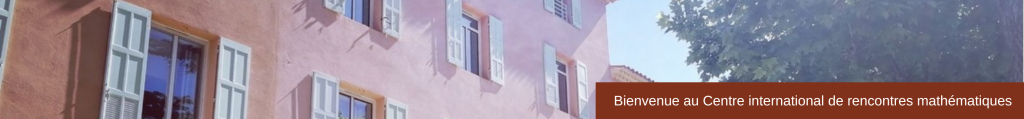## SLIDES

Square integrable representations, an invaluable tool: from coherent states to quantum mechanics on phase space   (pdf)

• Isiaka Aremua (Université de Lomé, Togo)

su (1,1) coherent states for Landau levels: Physical and mathematical description   (pdf)

Renormalization in spin foam quantum gravity with coherent states   (pdf)

Affine Coherent State Quantization and Quantum Cosmology   (pdf)

Lyapunov Theorem for continuous frames   (pdf)

New applications of coherent states in quantum information theory   (pdf)

Construction of linear and nonlinear coherent states using GHA   (pdf)

Regularised Bianchi IX potential   (pdf)

Phase retrieval in infinite dimensions   (pdf)

Entanglement of quantum circular states of light   (pdf)

SU (1,1) Covariant integral quantization of the unit disk   (pdf)

Coherent and minimum energy states of a charged particle in a uniform magnetic field   (pdf)

Coherent states, Support Vector Machines and function estimation   (pdf)

Wavelet Approximation Theory in Higher Dimensions   (pdf)

Minimal lenghts, areas and volumes in noncommutative quasi-Hermitian systems   (pdf)

• Katarzyna Górska (Polish Academy of Sciences)

Hermite polynomials in two complex variables Mathematical properties   ﻿(pdf)﻿

Non-Hermitian coherent states for finite-dimensional systems   (pdf)

Coherent state transforms for compact groups, and their large-N limits   (pdf)

Hermite polynomials in two complex variables   (pdf)

Coherent states for supersymmetric partners of solvable systems   ﻿(pdf)

Representations of CCR describing infinite coherent states   (pdf)﻿

Enhanced Quantization: The Right Way to Quantize Everything   (pdf)

Coherent states in a study of time problem   (pdf)

Two dimensional de Sitter spinors and their SL (2,R) covariance   (pdf)

Orthogonal polynomials attached to coherent states for the symmetric Pöschl-Teller oscillator   (pdf)

Classical and quantum Kummer shape algebras   (pdf)

Fermionic coherent states in infinite dimensions   (pdf)

Spacetime replication of continuous-variable quantum information   (pdf)

Higher order squeezing of noncommutative q-photon-added coherent states   (pdf)

Reproducing pairs and Gabor systems at critical density   (pdf)

Geometric aspects of coherent states   ﻿(pdf)﻿

The anatomy of coherent states   (pdf)

Coherent state quantization and the Heisenberg uncertainty relation in the quaternionic setting    (pdf)

Shearlets: Theory, applications and generalizations   (pdf)

Coherent spaces, Boolean rings and their applications   ﻿(pdf)﻿

Finite dimensional Hilbert space: Spin Coherent, Basis Coherent and Anti-coherent states   (pdf)